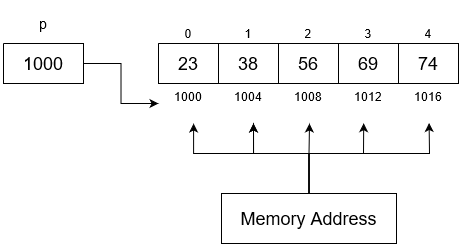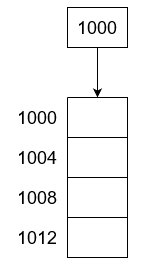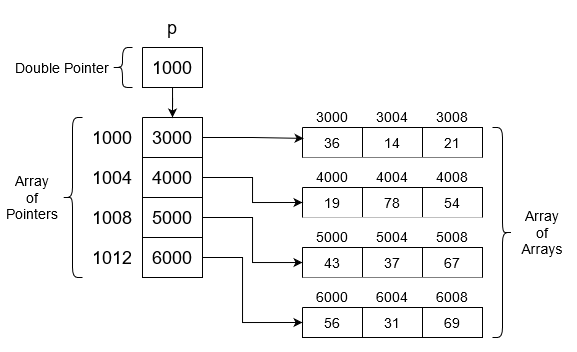# Creating array of pointers in C++

• Difficulty Level : Medium
• Last Updated : 04 Sep, 2021

An array of pointers is an array of pointer variables. It is also known as pointer arrays. We will discuss how to create a 1D and 2D array of pointers dynamically. The word dynamic signifies that the memory is allocated during the runtime, and it allocates memory in Heap Section. In a Stack, memory is limited but is depending upon which language/OS is used, the average size is 1MB.

Dynamic 1D Array in C++: An array of pointers is a type of array that consists of variables of the pointer type. It means that those variables can point to some other array elements.

Want to learn from the best curated videos and practice problems, check out the C++ Foundation Course for Basic to Advanced C++ and C++ STL Course for foundation plus STL.  To complete your preparation from learning a language to DS Algo and many more,  please refer Complete Interview Preparation Course.

Example:

int *p;

// Now P, P, P can point to int memory blocks.

In a dynamically allocated array of size N, the block is created in the heap and returns the address of the first memory block. By using that address every element can be accessed. The dynamic array in C++ one should be familiar with the new keywords or malloc(), calloc() can be used.

Syntax:

<dataType> * <pointer name> = new <dataType> [<size>];

Example:

int *p = new int ;Accessing Elements of a Dynamic Array:

• 1. 1D array of size N(= 5) is created and the base address is assigned to the variable P. If the below statement is written then the output is 1000.

cout << p;

• If the value in the 1000th address is wanted then dereferenced it using the * (asterisk) symbol as illustrated below:

cout << *P;

// It is the same as P. The output is 23.

Basic Pointer Arithmetic: Below is some points regarding Pointer Arithmetic:

• *(P + 1):

P = 1000 and 1 = sizeof(int) = 4 bytes.
Hence, *(1004) and dereferencing by * (asterisk) symbol. Now, the final result is 38.

• *(P) + 1:

P = 1000 and 1 = sizeof(int) = 4 bytes.
Hence, *(1004) and dereferencing by * (asterisk) symbol and then by adding 1 modifies the result to 23 + 1 = 24.

Below is the C++ program to illustrate the above concepts:

## C++

 `// C++ program to illustrate the concepts``// of creating 1D array of pointers``#include ``using` `namespace` `std;` `// Driver Code``int` `main()``{``    ``// Dynamically creating the array``    ``// of size = 5``    ``int``* p = ``new` `int``;` `    ``// Initialize the array p[] as``    ``// {10, 20, 30, 40, 50}``    ``for` `(``int` `i = 0; i < 5; i++) {``        ``p[i] = 10 * (i + 1);``    ``}` `    ``// Print the values using pointers``    ``cout << *p << endl;``    ``cout << *p + 1 << endl;``    ``cout << *(p + 1) << endl;``    ``cout << 2 [p] << endl;``    ``cout << p << endl;``    ``*p++;` `    ``// Pointing to next location``    ``cout << *p;` `    ``return` `0;``}`
Output
```10
11
20
30
30
20```

Dynamic 2D Array of Pointers in C++: A dynamic array of pointers is basically an array of pointers where every array index points to a memory block. This represents a 2D view in our mind. But logically it is a continuous memory block.

Syntax:

<dataType> **<Pointer name> = new <dataType> *[<size>];

Example:

int **P = new int *;

Note: The *(asterisk) symbol defines the level of the pointer, one * means one level of pointers, where ** implies two levels of pointers, and so on. Also, the level of the pointer must be the same as the dimensional array you want to create dynamically.

Approach:

• Create a 1D array of pointers.• Now, create the column as array of pointers for each row as:
• P = new int ;
• P = new int ;
• P = new int ;
• P = new int ;• The 1D array of pointers are pointing to a memory block(size is mentioned). Basically, P, …, P are pointing to a 1D array of integers.

Accessing the array elements:

• *P is equal to P which is the address of the 1st row, 1st column is &P = 3000.
• *(P + 1) is equal to ‘P‘ is 1000 + 1(sizeof int) = 1004 and * means dereferencing. So the value stored at the address is printed i.e., *1004 = 4000.
• *(P + 1) + 2 is same as above case but +2 means (&P + 2) is equal to &P  = 4008.
• *(*(P + 1) + 2) is same as above case but that first asterisk ‘*(….)’ means dereferencing that address. Therefore, the result is equal to the value in &P = *(4008) = 54.

Below is the C++ program to illustrate the above concepts:

## C++

 `// C++ program to illustrate the concepts``// of creating 2-D array of pointers``#include ``using` `namespace` `std;` `// Driver Code``int` `main()``{``    ``int` `N = 3;` `    ``// Creating the array of pointers``    ``// of size N``    ``int``** p = ``new` `int``*[N];``    ``int` `x = 1;` `    ``// For multiplying``    ``for` `(``int` `i = 0; i < N; i++) {` `        ``p[i] = ``new` `int``[N];` `        ``// Creating N sized int memory``        ``// block``        ``for` `(``int` `j = 0; j < N; j++, x++) {` `            ``p[i][j] = 10 * x;` `            ``// The above statement can``            ``// also be written as:``            ``// *(*(p+i)+j) = 10 * x``        ``}``    ``}` `    ``// Print the values using pointers``    ``cout << *p << endl;``    ``cout << **p << endl;``    ``cout << *p + 1 << endl;``    ``cout << **p + 1 << endl;``    ``cout << *(*(p + 1) + 0) << endl;``    ``cout << p << endl;` `    ``return` `0;``}`
Output
```0x158de90
10
0x158de94
11
40
90```

My Personal Notes arrow_drop_up